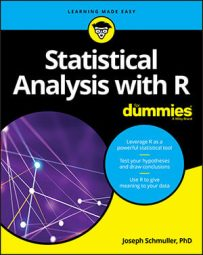##### Statistical Analysis with R For DummiesR provides a wide array of functions to help you with statistical analysis with R—from simple statistics to complex analyses. Several statistical functions are built into R and R packages. R statistical functions fall into several categories including central tendency and variability, relative standing, t-tests, analysis of variance and regression analysis.

## Base R statistical functions for central tendency and variability

Here’s a selection of statistical functions having to do with central tendency and variability that come with the standard R installation. You’ll find many others in R packages.

Each of these statistical functions consists of a function name immediately followed by parentheses, such as `mean()`, and `var()`. Inside the parentheses are the arguments. In this context, “argument” doesn’t mean “disagreement,” “confrontation,” or anything like that. It’s just the math term for whatever a function operates on.

 Function What it Calculates `mean(x)` Mean of the numbers in vector x. `median(x)` Median of the numbers in vector x `var(x)` Estimated variance of the population from which the numbers in vector x are sampled `sd(x)` Estimated standard deviation of the population from which the numbers in vector x are sampled `scale(x)` Standard scores (z-scores) for the numbers in vector x

## Base R Statistical Functions for Relative Standing

Here’s a selection of R statistical functions having to do with relative standing.

Function What it Calculates
`sort(x)` The numbers in vector x in increasing order
`sort(x)[n]` The nth smallest number in vector x
`rank(x)` Ranks of the numbers (in increasing order) in vector x
`rank(-x)` Ranks of the numbers (in decreasing order) in vector x
`rank(x, ties.method= “average”)` Ranks of the numbers (in increasing order) in vector x, with tied numbers given the average of the ranks that the ties would have attained
 `rank(x, ties.method=  “min”)`
Ranks of the numbers (in increasing order) in vector x, with tied numbers given the minimum of the ranks that the ties would have attained
`rank(x, ties.method = “max”)` Ranks of the numbers (in increasing order) in vector x, with tied numbers given the maximum of the ranks that the ties would have attained
`quantile(x)` The 0th, 25th, 50th, 75th, and 100th percentiles (i.e, the quartiles) of the numbers in vector x. (That’s not a misprint: quantile(x) returns the quartiles of x.)

## T-Test Functions for Statistical Analysis with R

Here’s a selection of R statistical functions having to do with t-tests.

 Function What it Calculates `t.test(x,mu=n, alternative = “two.sided”)` Two-tailed t-test that the mean of the numbers in vector x is different from n. `t.test(x,mu=n, alternative = “greater”)` One-tailed t-test that the mean of the numbers in vector x is greater than n. `t.test(x,mu=n, alternative = “less”)` One-tailed t-test that the mean of the numbers in vector x is less than n. `t.test(x,y,mu=0, var.equal  = TRUE, alternative = “two.sided”)` Two-tailed t-test that the mean of the numbers in vector x is different from the mean of the numbers in vector y. The variances in the two vectors are assumed to be equal. `t.test(x,y,mu=0, alternative = “two.sided”, paired  = TRUE)` Two-tailed t-test that the mean of the numbers in vector x is different from the mean of the numbers in vector y. The vectors represent matched samples.

## ANOVA and Regression Analysis Functions for Statistical Analysis with R

Here’s a selection of R statistical functions having to do with Analysis of Variance (ANOVA) and correlation and regression.

When you carry out an ANOVA or a regression analysis, store the analysis in a list. For example,

`a <- lm(y~x, data = d)`

Then, to see the tabled results, use the summary() function:

`summary(a)`

 Function What it Calculates `aov(y~x, data = d)` Single-factor ANOVA, with the numbers in vector y as the dependent variable and the elements of vector x as the levels of the independent variable. The data are in data frame d. `aov(y~x + Error(w/x), data = d)` Repeated Measures ANOVA, with the numbers in vector y as the dependent variable and the elements in vector x as the levels of an independent variable. Error(w/x) indicates that each element in vector w experiences all the levels of x (i.e., x is a repeated measure). The data are in data frame d. `aov(y~x*z, data = d)` Two-factor ANOVA, with the numbers in vector y as the dependent variable and the elements of vectors x and z as the levels of the two independent variables. The data are in data frame d. `aov(y~x*z + Error(w/z), data = d)` Mixed ANOVA, with the numbers in vector z as the dependent variable and the elements of vectors x and y as the levels of the two independent variables. Error(w/z) indicates that each element in vector w experiences all the levels of z (i.e., z is a repeated measure). The data are in data frame d.
 Function What it Calculates `cor(x,y)` Correlation coefficient between the numbers in vector x and the numbers in vector y `cor.test(x,y)` Correlation coefficient between the numbers in vector x and the numbers in vector y, along with a t-test of the significance of the correlation coefficient. `lm(y~x, data = d)` Linear regression analysis with the numbers in vector y as the dependent variable and the numbers in vector x as the independent variable. Data are in data frame d. `coefficients(a)` Slope and intercept of linear regression model a. `confint(a)` Confidence intervals of the slope and intercept of linear regression model a `lm(y~x+z, data = d)` Multiple regression analysis with the numbers in vector y as the dependent variable and the numbers in vectors x and z as the independent variables. Data are in data frame d.